# How to Find the Distance Between Two Points with Given Latitude and Longitude in PHP? - Geodesy PHP package blog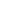All package blogs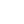Geodesy PHPGeodesy PHP package blog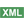How to Find the Dista...Post a comment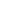See comments (1) Trackbacks (0)

Author:

Viewers: 1,199

Last month viewers: 113

Package: Geodesy PHP

Many applications require to find the distance between places on Earth.

This is a common problem that only requires knowing the geographic coordinates of those points to be solved.

Read this short article to learn how to solve this problem with a small effort using the Geodesy PHP package.

Geodesy-PHP is a PHP port of some known geodesic functions for getting distance from a known point A to a known point B, given their latitude and longitude (good for working out distances between latitude/longitude data provided by Google Maps or any Restful APIs).By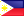<email contact>

## What is the GeoDesy PHP package?

Geodesy-PHP is a PHP implementation of some well-known geodesic functions for getting the distance from a known point A to a known point B, given their latitude and longitude.

This is a good solution when you are trying to find out what are the distances between locations on Earth given the latitude and longitude coordinate values data provided by Google Maps or any Restful APIs.

This has been helpful to me at a time when working out the distance between different vendors of certain products or services and the user's location, as well check if they are within a located within a certain distance range.

It provides different methods for calculating distances implemented by different classes in this package:

### How to Use GeoDesy PHP to Calculate the Location Distances?

Both HaversineFormula and SphericalCosine Classes returns results in kilometers. While the VincentyFormula is more accurate and returns the distances in meters.

Here follows a simple example code sample of how to use these classes:

```use Geodesy\Location\LatLong;
use Geodesy\Distance\HaversineFormula;
use Geodesy\Distance\VincentyFormula;
use Geodesy\Distance\SphericalCosine;

\$lat1 = 48.148636;
\$long1 = 17.107558;

\$lat2 = 48.208810;
\$long2 = 16.372477;

\$loc1 = new LatLong;
\$loc1->setLatitude(\$lat1);
\$loc1->setLongitude(\$long1);

\$loc2 = new LatLong;
\$loc2->setLatitude(\$lat2);
\$loc2->setLongitude(\$long2);

// SphericalCosine(\$loc1, \$loc2) or VincentyFormula(\$loc1, \$loc2)
\$distance = new HaversineFormula(\$loc1, \$loc2);

// get the distance
\$distance->getDistance();

/**
* Is within range?
* Haversine and Cosine both assumes the value as kilometer/s while VincentyFormula is in meter/s.
*/
if(\$distance->isInRange(500)) {
print_r('yehey! your lost friend is near you..');
}
```

## Conclusion

As you may notice, the GeoDesy PHP package makes it very simple to perform calculations of distances between two locations on the planet using methods that provide different approaches to calculate distances with greater accuracy if necessary.

Feel free to comment below if you have questions and please help sharing this article if you liked it and know that it will be useful for others to know about these solutions to find out distances between two locations on Earth.

## 1,584,389 PHP developers registered to the PHP Classes site. Be One of Us!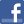Facebook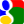Gmail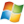Hotmail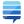StackOverflow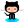GitHub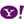YahooPost a commentSee comments (1) Trackbacks (0)All package blogsGeodesy PHPGeodesy PHP package blogHow to Find the Dista...Advertise on this siteSite mapNewsletterStatisticsSite tipsPrivacy policyContact
For more information send a message to `info at phpclasses dot org`.